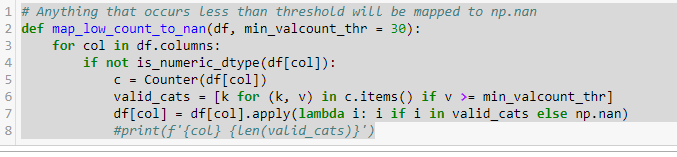# Is there a function in fastai to restrict the number of categorical levels based on frequency

For an experiment I wanted to map all the low count categorical values to ‘UNKNOWN’ or level 0 by default. I looked into the following function codes and it seems like it is not supported. This is to avoid create embedding for categorical values which have low frequency. Low frequency categorical values may not get sufficient updates to get trained accurately. Is this already supported?

Anyway I can do preprocessing of the dataframe categorical values to do this.

Functions I looked into:

def proc_df(df, y_fld, skip_flds=None, do_scale=False, na_dict=None,
preproc_fn=None, max_n_cat=None, subset=None):

def numericalize(df, col, name, max_n_cat):
if not is_numeric_dtype(col) and ( max_n_cat is None or col.nunique()>max_n_cat):
df[name] = col.cat.codes+1

It’s a good idea. I think you need to do this manually - if you come up with a nice method, I’d be happy to add it to fastai

Thanks Jeremy.

I was thinking of using following approach suggested in stackoverflow:

Replace all values with value_counts below a threshold to np.nan. This will automatically make sure that train_cats will set them to zero.

Sample code:
df = pd.DataFrame(np.random.randint(0, high=9, size=(100,2)),
columns = [‘A’, ‘B’])

df = df.astype(str)

threshold = 10 # Anything that occurs less than this will be removed.
for col in df.columns:
value_counts = df[col].value_counts() # Specific column
to_remove = value_counts[value_counts <= threshold].index
df[col].replace(to_remove, np.nan, inplace=True)

train_cats(df)

Yeah that’s the kind of thing I was thinking…

I finally wrote this function for my purpose. Faced some performance issues with earlier example using value_countsExcellent - maybe post it in a code block here, rather than a pic, so others can use it more easily?

``````def map_low_count_to_nan(df, min_valcount_thr=30):
for col in df.columns:
if not is_numeric_dtype(df[col]):
c = Counter(df[col])
valid_cats=[k for (k,v) in c.items() if v >= min_valcount_thr]
df[col] = df[col].apply(lambda i: i if i in valid_cats else np.nan)

``````